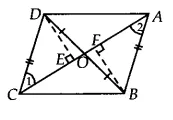Q

# In Fig. 9.25, diagonals AC and BD of quadrilateral ABCD intersect at O such that OB = OD. If AB = CD, then show that: (ii) ar (DCB) = ar (ACB)

Q : 6     In Fig. , diagonals AC and BD of quadrilateral ABCD intersect at O such that . If , then show that:

(ii)

[Hint: From D and B, draw perpendiculars to AC.]Views